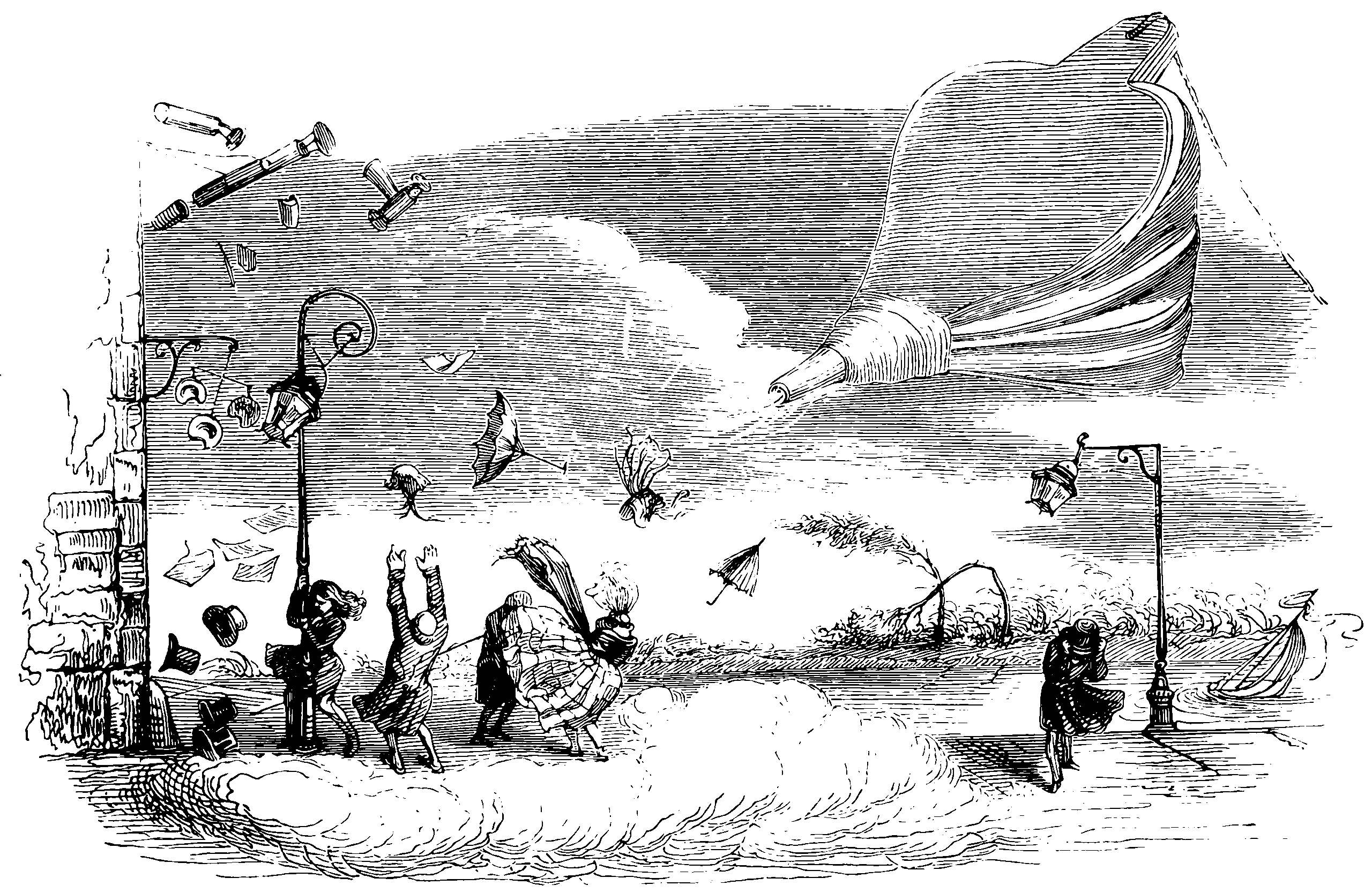# Bayesian posterior inference via optimisationRandomly exploring the posterior space.

The Bayes-by-backprop terminology seems to come from Blundell et al. (2015).

## Bayesian learning rule

M. E. Khan and Rue (2022):

We show that a wide-range of well-known learning-algorithms from a variety of fields are all specific instances of a single learning algorithm derived from Bayesian principles. The starting point, is the variational formulation by Zellner (1988), which is an extension of [Eq. 1 to optimize over a well-defined candidate distribution $$q(\boldsymbol{\theta})$$, and for which the minimizer $q_*(\boldsymbol{\theta})=\underset{q(\boldsymbol{\theta})}{\arg \min } \quad \mathbb{E}_q\left[\sum_{i=1}^N \ell\left(y_i, f_{\boldsymbol{\theta}}\left(\boldsymbol{x}_i\right)\right)\right]+\mathbb{D}_{K L}[q(\boldsymbol{\theta}) \| p(\boldsymbol{\theta})]$ defines a generalized posterior in lack of a precise likelihood. The prior distribution is related to the regularizer, $$p(\boldsymbol{\theta}) \propto \exp (-R(\boldsymbol{\theta}))$$, and $$\mathbb{D}_{K L}[\cdot \| \cdot]$$ is the Kullback-Leibler Divergence (KLD). In the case where $$\exp \left(-\ell\left(y_i, f_{\boldsymbol{\theta}}\left(\boldsymbol{x}_i\right)\right)\right)$$ is proportional to the likelihood for $$y_i, \forall i$$, then $$q_*(\boldsymbol{\theta})$$ is the posterior distribution for $$\boldsymbol{\theta}$$

The result is heavy on natural gradient and exponential families. Also Emti is very charismatic and I defy you to watch his presentation and not feel like this is the One True Way, at least for a few minutes. rPRobably related: Knoblauch, Jewson, and Damoulas (2022).

## SGD as MCMC

Combining Markov Chain Monte Carlo and Stochastic Gradient Descent for in the sense of using SGD to do some cheap approximation to MCMC posterior sampling. Overviews in Ma, Chen, and Fox (2015) and Mandt, Hoffman, and Blei (2017). A lot of probabilistic neural nets leverage this idea.

A related idea is estimating gradients of parameters by Monte Carlo; there is nothing necessarily Bayesian about that per se; in that case we are doing a noisy estimate of a deterministic quantity. In this setting we are interested in the noise itself.

I have a vague memory that this argument is leveraged in Neal (1996)? Should check. For sure the version in Mandt, Hoffman, and Blei (2017) is a highly developed and modern take. Basically, they analyse the distribution near convergence as an autoregressive process:

Stochastic Gradient Descent with a constant learning rate (constant SGD) simulates a Markov chain with a stationary distribution. With this perspective, we derive several new results.

1. We show that constant SGD can be used as an approximate Bayesian posterior inference algorithm. Specifically, we show how to adjust the tuning parameters of constant SGD to best match the stationary distribution to a posterior, minimizing the Kullback-Leibler divergence between these two distributions.
2. We demonstrate that constant SGD gives rise to a new variational EM algorithm that optimizes hyperparameters in complex probabilistic models.
3. We also propose SGD with momentum for sampling and show how to adjust the damping coefficient accordingly.
4. We analyze MCMC algorithms. For Langevin Dynamics and Stochastic Gradient Fisher Scoring, we quantify the approximation errors due to finite learning rates. Finally,
5. we use the stochastic process perspective to give a short proof of why Polyak averaging is optimal. Based on this idea, we propose a scalable approximate MCMC algorithm, the Averaged Stochastic Gradient Sampler.

The article is rather beautiful. Importantly they leverage the assumption that we are sampling from approximately (log-)quadratic posterior modes, which means that we should be suspicious of the method when

1. The posterior is not quadratic, i.e. the distribution is not well approximated by a Gaussian at the mode, and
2. The same for the tails. If there are low-probability but high importance posterior configurations such that they are not Gaussian in the tails, we should be skeptical that they will be sampled well; I have an intuition that this is a more stringent requirement, but TBH I am not sure of the exact relationship of these two conditions.

The analysis leverages gradient flow, which is a continuous limit of stochastic gradient descent.

## Stochastic Weight Averaging

A popular recent development is the Stochastic Weight Averaging family of methods . See Andrew G Wilson’s web page for a brief description of the sub methods, since he seems to have been involved in all of them.

## Stein Variational GD

Perhaps related? An ensemble method. See Stein VGD.

## SG Hamiltonian Monte Carlo

This, surprisingly, works, I am told? T. Chen, Fox, and Guestrin (2014).

## SG thermostats

Some kind of variance control using auxiliary variables? See Ding et al. (2014).

## SG Fisher scoring

See Ahn, Korattikara, and Welling (2012). I assume there is a connection to MC gradients via the score trick?.

### No comments yet. Why not leave one?

GitHub-flavored Markdown & a sane subset of HTML is supported.